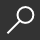## Fundamentals of Diophantine Geometry by S. Lang

Overview -
Diophantine problems represent some of the strongest aesthetic attractions to algebraic geometry. They consist in giving criteria for the existence of solutions of algebraic equations in rings and fields, and eventually for the number of such solutions. The fundamental ring of interest is the ring of ordinary integers Z, and the fundamental field of interest is the field Q of rational numbers. One discovers rapidly that to have all the technical freedom needed in handling general problems, one must consider rings and fields of finite type over the integers and rationals. Furthermore, one is led to consider also finite fields, p-adic fields (including the real and complex numbers) as representing a localization of the problems under consideration. We shall deal with global problems, all of which will be of a qualitative nature. On the one hand we have curves defined over say the rational numbers. Ifthe curve is affine one may ask for its points in Z, and thanks to Siegel, one can classify all curves which have infinitely many integral points. This problem is treated in Chapter VII. One may ask also for those which have infinitely many rational points, and for this, there is only Mordell's conjecture that if the genus is: 2, then there is only a finite number of rational points.

local_shippingFor DeliveryIn Stock.
This item is Non-Returnable.
FREE Shipping for Club Members helpNew & Used Marketplace 4 copies from \$35.00

## More About Fundamentals of Diophantine Geometry by S. Lang

### Overview

Diophantine problems represent some of the strongest aesthetic attractions to algebraic geometry. They consist in giving criteria for the existence of solutions of algebraic equations in rings and fields, and eventually for the number of such solutions. The fundamental ring of interest is the ring of ordinary integers Z, and the fundamental field of interest is the field Q of rational numbers. One discovers rapidly that to have all the technical freedom needed in handling general problems, one must consider rings and fields of finite type over the integers and rationals. Furthermore, one is led to consider also finite fields, p-adic fields (including the real and complex numbers) as representing a localization of the problems under consideration. We shall deal with global problems, all of which will be of a qualitative nature. On the one hand we have curves defined over say the rational numbers. Ifthe curve is affine one may ask for its points in Z, and thanks to Siegel, one can classify all curves which have infinitely many integral points. This problem is treated in Chapter VII. One may ask also for those which have infinitely many rational points, and for this, there is only Mordell's conjecture that if the genus is: 2, then there is only a finite number of rational points.

This item is Non-Returnable.

### Details

• ISBN-13: 9780387908373
• ISBN-10: 0387908374
• Publisher: Springer
• Publish Date: August 1983
• Page Count: 370
• Dimensions: 9.21 x 6.14 x 0.88 inches
• Shipping Weight: 1.59 poundsRelated Categories